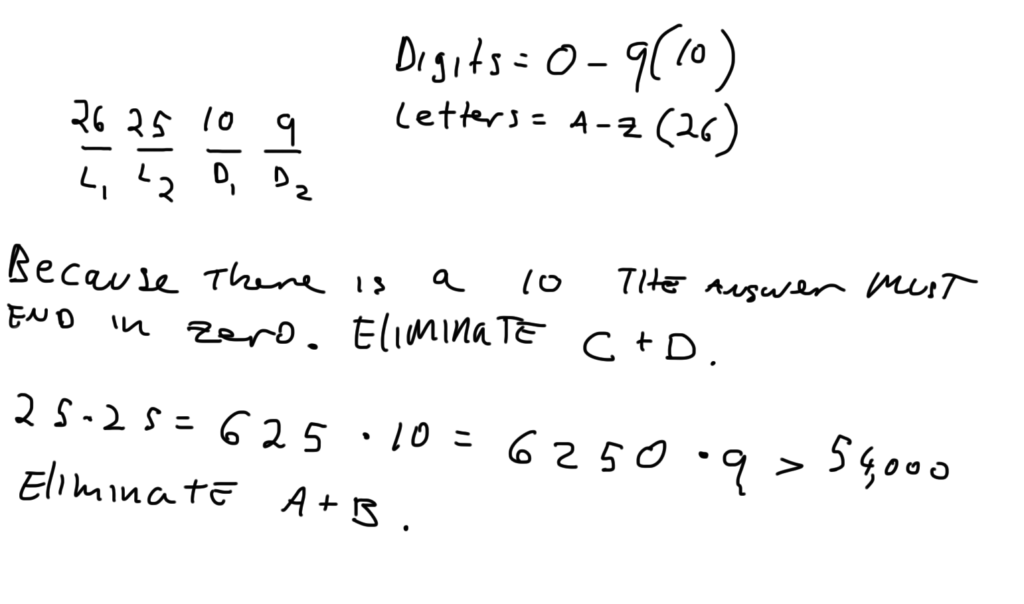Private GMAT Tutoring in NYC & Online

# GMAT Question of the Day - Problem Solving - Combinations/Permutations

An animal shelter is producing unique tags for each of the x number of animals at the shelter. Each tag will have a code made up of two letters and two digits with no letters or digits repeating. If there are exactly the same number of animals as there are codes then what is the value of x?

A. 46430

B. 48690

C. 52864

D. 56862

E. 58500

## GMAT Question of the Day Solution:

In this question we're being asked to make codes. That means the order matters. This is the same as figuring out how many different ways you can line up a group of children, how many ways a group of people can walk through a door, or how many combinations you can make for a lock given a certain number of numbers. The slot method works well for this. Also, you've got some pretty big numbers and therefore some potentially long calculations. Look for ways to simplify your work. There is a 10 in the multiplication so the answer must end in 0. You can also do some rough arithmetic using easy calculations (25*25*10*9) and set a lower limit for the answer. See the diagram below:# CONTACT

Atlantic GMAT

405 East 51st St.

NY, NY 10022

(347) 669-3545

info@AtlanticGMAT.com